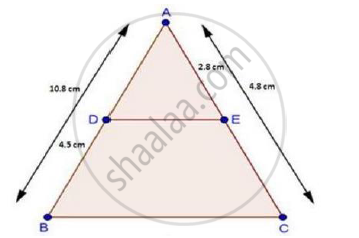Share

Books Shortlist
Your shortlist is empty

# Solution for In a δAbc, D and E Are Points on the Sides Ab and Ac Respectively. For the Following Case Show that De || Bc Ab = 10.8 Cm, Bd = 4.5 Cm, Ac = 4.8 Cm and Ae = 2.8 Cm. - CBSE Class 10 - Mathematics

ConceptBasic Proportionality Theorem Or Thales Theorem

#### Question

In a ΔABC, D and E are points on the sides AB and AC respectively. For  the following case show that DE || BC

AB = 10.8 cm, BD = 4.5 cm, AC = 4.8 cm and AE = 2.8 cm.

#### SolutionWe have,

AB = 10.8cm, BD = 4.5cm, AC = 4.8 cm and AE = 2.8cm

∴ AD = AB – DB = 10.8 – 4.5

⇒ AD = 6.3 cm

And, EC = AC – AE

= 4.8 – 2.8

⇒ EC = 2 cm

Now, "AD"/"DB"=6.3/4.5=7/5        [∵ AD = 6.3 cm]

And, "AE"/"EC"=2.8/2=28/20=7/5      [∵ EC = 2 cm]

Thus, DE divides sides AB and AC of ΔABC in the same ratio.

Therefore, by the converse of basic proportionality theorem.

We have, DE || BC

Is there an error in this question or solution?

#### Video TutorialsVIEW ALL 

Solution for question: In a δAbc, D and E Are Points on the Sides Ab and Ac Respectively. For the Following Case Show that De || Bc Ab = 10.8 Cm, Bd = 4.5 Cm, Ac = 4.8 Cm and Ae = 2.8 Cm. concept: Basic Proportionality Theorem Or Thales Theorem. For the course CBSE
S